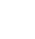# Zest Codes

``` 'This code looks down Sheet1 column A and considers the number of csv strings in column B and Column D 'Then puts that many times down the Output tab the code from Col A of that row.```

``` 'It then runs through the Output Tab and does the same except puts it in Output2 'so that we get all the permutations of the product Dim arr() As String OTP = 2 With Worksheets("Sheet1") lastrowSheet1 = .Cells(.Rows.Count, "A").End(xlUp).Row End With For n = 2 To lastrowSheet1 item_code = Worksheets("Sheet1").Range("A" & n) arr = Split(Worksheets("Sheet1").Range("B" & n).Value, ",") For i = LBound(arr) To UBound(arr) Worksheets("Output").Range("A" & OTP) = item_code Worksheets("Output").Range("B" & OTP) = arr(i) OTP = OTP + 1 Next Next n With Worksheets("Output") lastrowOutput = .Cells(.Rows.Count, "A").End(xlUp).Row End With For otp1 = 2 To lastrowOutput Worksheets("Output").Range("C" & otp1) = WorksheetFunction.VLookup(Worksheets("Output").Range("A" & otp1), Worksheets("Sheet1").Range("A:Z"), 3, False) Worksheets("Output").Range("D" & otp1) = WorksheetFunction.VLookup(Worksheets("Output").Range("A" & otp1), Worksheets("Sheet1").Range("A:Z"), 4, False) Next otp1 OTP = 2 For n = 2 To lastrowOutput Application.StatusBar = n item_code = Worksheets("Output").Range("A" & n) arr = Split(Worksheets("Output").Range("D" & n).Value, ",") For i = LBound(arr) To UBound(arr) Worksheets("Output2").Range("A" & OTP) = Trim(item_code) Worksheets("Output2").Range("B" & OTP) = Trim(Worksheets("Output").Range("B" & n)) Worksheets("Output2").Range("C" & OTP) = Trim(Worksheets("Output").Range("C" & n)) Worksheets("Output2").Range("D" & OTP) = Trim(arr(i)) OTP = OTP + 1 Next Next n 'So far we've put all the permutations of the items branding and colours in to a master list. 'We now need to populate all the costs 'Item price - where do we get the item price from ? 'Is it the lowest price at the right of the item price tables or the first price it comes to ? 'Loop down Output2 and grab the item_code (we will grab and check each one many times) With Worksheets("Output2") lastrowOutput2 = .Cells(.Rows.Count, "A").End(xlUp).Row End With For m = 2 To lastrowOutput2 item_code = Worksheets("Output2").Range("A" & m) 'New we've got the item number, lets grab the item price from the price page With Worksheets("Prices") lastrowPrices = .Cells(.Rows.Count, "A").End(xlUp).Row End With For p = 2 To lastrowPrices 'Assume the item code is in column A If item_code = Worksheets("Prices").Range("A" & p) Then item_row = p Exit For End If Next p 'Now we have the row for the item, find the price '************************************************************************************************************* '************************************************************************************************************* '************************************************************************************************************* 'We have to choose whether to take the left or the rightmost price below but we also have to decide if there is 'a premium to add to the item price by a code given by the manufacturer item_premium_code = Worksheets("Output2").Range("X" & m) item_premium = 0 'If item_premium_code = a Then item_premium = Worksheets("Premiums").Range("X25") 'Leftmost price item_price = 0 For c = 4 To 8 'Columns D to H which we can expand if required If Worksheets("Prices").Cells(p, c) <> "" Then item_price = Worksheets("Prices").Cells(p, c) Exit For End If Next c ''''''Rightmost price '''''item_price = 0 ''''' '''''For c = 8 To 4 Step -1 'Columns H to D which we can expand if required '''''If Worksheets("Prices").Cells(p, c) <> "" Then '''''item_price = Worksheets("Prices").Cells(p, c) '''''Exit For '''''End If '''''Next c '************************************************************************************************************* '************************************************************************************************************* '************************************************************************************************************* 'Whichever we have chosen, leftmost or rightmost, we now have the item price 'x is the range of columns where item_price is going For x = 10 To 31 Worksheets("Output2").Cells(m, x) = WorksheetFunction.Sum(Val(item_price) + Val(item_premium)) Next x Next m ```